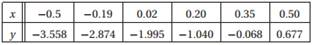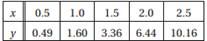### Create an Account

Home / Questions / 1 Determine a and b for which fx a sinπx2 bcosπx2 fits the following data in the least s...

# 1 Determine a and b for which fx a sinπx2 bcosπx2 fits the following data in the least squares sense 2 Determine a and b so that

1. Determine a and b for which f(x) = a sin(πx/2) + bcos(πx/2) fits the following data in the least-squares sense.2. Determine a and b so that f(x) = axb fits the following data in the least-squares sense.Jul 27 2020 View more View LessSubscribe To Get Solution